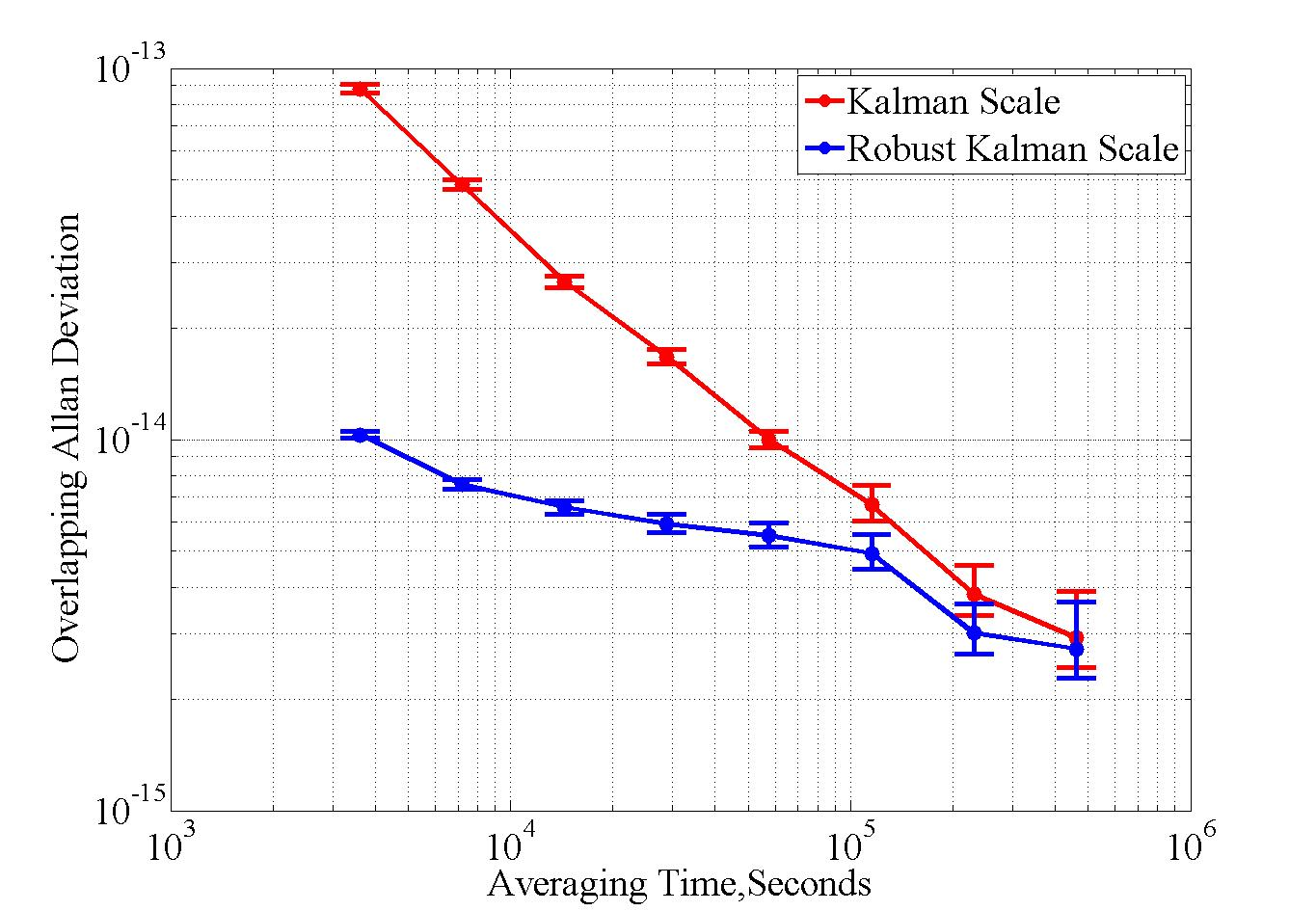Research Progress

# Researchers Improved Kalman Filter Time Scale Algorithm

Author：SONG Huijie       ArticleSource：       Update time：2021/07/12

Recently, Researchers from Time-keeping Laboratory of the National Time Service Centre of The Chinese Academy of Sciences (CAS) improved Kalman filter time scale algorithm under the condition of abnormal atomic clock data.

Kalman filter time scale algorithm is a common method in time generation and time-keeping work. However, The abnormal atomic clock data could disturb the estimation of Kalman filter time scale algorithm, reducing the accuracy and stability of time scale.

Researchers led equivalent covariance matrix and adaptive factor into the Kalman filter time scale algorithm. The equivalent covariance matrix is constructed to control the influence of the measurement outliers on the state estimation of the atomic clock, and the growth of the state prediction covariance matrix is controlled by the real-time calculation of the adaptive factor with statistics, reducing the influence of the atomic clock outliers on the state estimation.

In this study, researchers analyzed Kalman filter algorithm for abnormal atomic clock data from simulated and test data and compared with the classical Kalman filter algorithm. The results show that the improved Kalman filter algorithm can effectively resist the influence of abnormal data on the time scale, and improve the accuracy and stability of time scale when the abnormal data occur. The study also analyzed algorithm performance of non-Gaussian noise, and compared the least square threshold which set for abnormal date processing. The results suggest that the improved Kalman filter is better than the least square pretreatment in the stability of the computation time scale.

The results published in Journal of Instrumentation (Volume 16,June 2021 JINST 16 P06032 ) as the title: A robust Kalman filter time scale algorithm with data anomaly.Figure 1. Time scale stability comparison based on the two Kalman filter algorithms.(Image by NTSC)Figure2. The corrected time deviations of atomic clock based on the two algorithms. (Image by NTSC)

Appendix(es)：